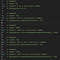# Algo Breakdown — Narcissistic Number

Hey all, I’m back at it with another algorithm breakdown. This is a format I’ve done before so if you like it you can check out a similar article I published here, leave a comment, or connect on LinkedIn. I have a lot of fun figuring out and breaking down problems so it’s always a pleasure to hear other perspectives. Anyway, enough on that, let’s get to it.

In this problem, we have to write an algorithm that can check if the integer input is a narcissistic number. Narcissistic numbers are whole numbers, or integers, whose digits each to the nth power sum to equal the whole number or integer itself, where nth is the number of digits.
You can find a list of Narcissistic numbers here.The exponent of each digit is equal to the number of digits

My initial thought to solving this algorithm is to first split the integer input into an array of integers. In Javascript, this can be done with the Array.from() method, as demonstrated here. I’ll store the result in a variable digitArray.

Next, I would like to iterate through digitArray finding the nth power of each element where ’n’ is the length of the array (which is the same as the number of digits in our input integer). I’ll take each total and add it to a variable that will track the sum of the numbers in our digitArray as we iterate through so we can compare it with our original input later. We can use Math.pow() to find exponents in Javascript.

Lastly, after our loop breaks, we can compare our input with comparisonNum and conditional return a message telling us whether or not the input is a narcissistic number.

Then we can clean it up a bit to make it presentable.

To test this solution I ran the following tests: